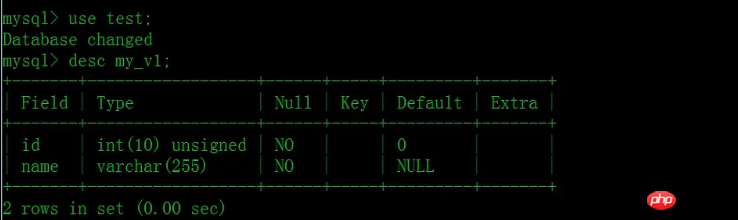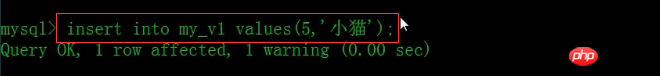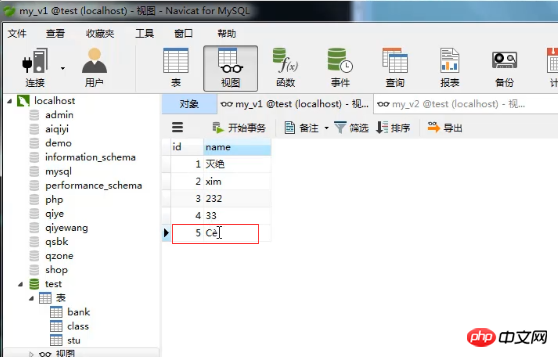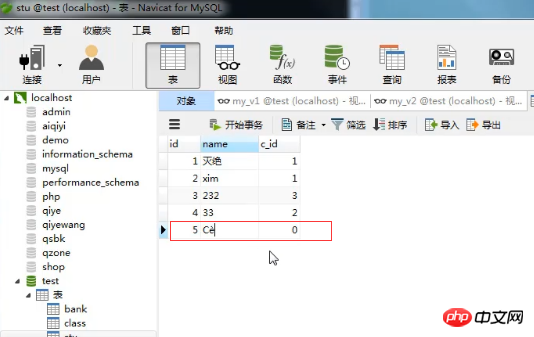•5星
10.46MB xipengbozai 2021-03-22 00:43:45
•5星
91.43MB daokedream 2021-02-09 15:30:35
•5星
270KB evanyanglibo 2021-02-23 17:05:37
•5星
67.5MB qq_43552032 2020-12-30 21:40:56
•5星
483KB qq_46051303 2021-01-21 14:17:39
•5星
21.37MB weixin_43959833 2021-03-27 15:06:27
•5星
12.98MB jkol12 2021-10-03 16:06:46
•5星
7.62MB r081r096 2021-01-19 01:30:38
• 另一个问题就是插入图表之后，就不能调出数据进行编辑，但是任务管理器里看到excel个进程的。这个两个问题是同步出现，过去一直以为是word本身的问题，一直等到微软出新的word2013补丁，安装到今天还是老样子，...访问量:

12

word2013使用起来很快，效果很好，可是发觉一个问题，word2013在win7和win8上老出一个问题，就是插入图表后，无法直接保存，保存点之后还是不能；另一个问题就是插入图表之后，就不能调出数据进行编辑，但是在任务管理器里看到有excel个进程的。这个两个问题是同步出现，过去一直以为是word本身的问题，一直等到微软出新的word2013补丁，安装到今天还是老样子，偶然的机会解决了这个问题。

一、出现以下主要症状(1)无法调出编辑框编辑数据(2)点击保存之后无法保存数据(3)在任务管理器中能看到excel这个进程存在

二、解决办法

找到Normal.dotm,并重新命名，就OK了，然后重开word暂时一切OK了。

为什么说暂时呢？因为根据微软官方说法:如果 Normal.dotm 被重命名、损坏或移走，Word 将在下次启动时自动创建新的版本(使用原始的默认设置)。新版本中将不包含您对已重命名或移走的版本所做过的任何自定义设置。(1)找到Normal.dotm(2)暂时正常演示

~谢谢打赏~展开全文weixin_42134537 2021-01-12 13:58:01
• mysql视图的相关知识我们之前的文章，都已经给大家详细介绍过了，相信大家对视图肯定了更深一步的了解。从前面文章的介绍，大家应该都知道了视图就是由SELECT语句组成的查询定义的虚拟表，是由一张或多张表...

本篇文章主要给大家介绍mysql数据表中视图是怎么新增数据的。

mysql视图的相关知识在我们之前的文章中，都已经给大家详细介绍过了，相信大家对视图肯定有了更深一步的了解。

从前面文章的介绍中，大家应该都知道了视图就是由SELECT语句组成的查询定义的虚拟表，是由一张或多张表中的数据组成的，从数据库系统外部来看，视图就如同一张数据表一样，对表能够进行的一般操作都可以应用于视图，比如查询，插入数据，修改视图，删除视图等等操作。

当我需要在视图中插入新的数据时，该怎么去实现插入新数据的操作呢？

下面我们就结合简单的示例，给大家介绍在单表视图中插入新数据的方法。

首先我们可以使用命令行工具查询视图中的字段信息。

查询字段信息的SQL语法：desc + 视图名 ;如图，my_v1这张视图中只有两个字段id，name。

然后我们在my_v1视图中插入一条新数据，SQL语句如下：如图我们使用insert into 语句向my_v1视图中插入了一个新的id和名称的数据。

我们再通过Navicat查看下数据是否插入成功。如图my_v1视图中数据已插入成功，不过由于编码问题“小猫”字段不能正常显示，这里就先不做详细介绍了。后期文章中会为大家统一详解mysql乱码的问题。

其实当我们给单表视图插入数据的同时，基表也就是组成这个视图的数据表中也会被插入同样的数据。注意：使用INSERT语句进行插入操作的视图必须能够在基表(组成视图查询的表)中插入数据，否则会操作失败。当我们给数据基本表插入新数据时，视图也会同步插入数据。

并且不能在一个语句中对多个基础表使用数据修改语句。所以如果要向一个引用了多个数据表的视图添加数据时，必须使用多个INSERT语句进行添加。

本篇文章就是关于给mysql视图增加新数据的方法介绍，也是非常简单易懂的， 希望对需要的朋友有所帮助！

想要了解更多mysql知识，可以关注PHP中文网mysql视频教程，欢迎大家参考学习！

展开全文weixin_36035610 2021-01-26 22:47:43
• 要在数据可视化中脱颖而出，你必须了解的 8 个图表用法 python 数据可视化 可视化

数据可视化主要旨在借助于图形化手段，清晰有效地传达与沟通信息，它与信息图形、信息可视化、科学可视化以及统计图形密切相关。当前，研究、教学和数据开发... 本主题，我们将使用 Python 介绍各种类型的图表

数据可视化主要旨在借助于图形化手段，清晰有效地传达与沟通信息，它与信息图形、信息可视化、科学可视化以及统计图形密切相关。

当前，在研究、教学和数据开发领域，数据可视化是一个极为活跃而又关键的方面。现代企业使用各种可视化技术来理解数据并从中获得洞察力，从而做出数据驱动的业务决策。也有很多可视化工具可用，例如 Tableau、Power BI、Looker、Qlik Sense 等等。

在本文中，我将分享8种常用的 Python 可视化图表，介绍它们的最佳使用场景，同时分享一个实战案例，欢迎大家收藏学习，喜欢点赞支持。文末提供技术交流群，欢迎畅聊。

数据可视化的必要性

当数据以简单的可视化的格式呈现时，数据更有意义并且更容易理解，因为人眼很难从原始数据中解读模式、趋势和季节性，因此，数据被可视化以了解不同参数的行为。

各种类型的图表及其用途1. 条形图和柱形图

这是了解我们的定量领域在各种类别中的表现的最简单的图表之一，它用于比较。从上面的柱状图可以看出，技术类的销售额较高，办公用品的销售额最少。上图是一个条形图，显示 L 类别的表现更好。

2. 散点图和气泡图

散点图和气泡图帮助我们了解变量如何分布在所考虑的范围内。 它可用于识别模式、异常值的存在以及两个变量之间的关系。我们可以看到，随着折扣的增加，利润也在减少。3. 折线图

当必须呈现与时间相关的数据时，它是首选。 它最适合分析趋势。从上图中，我们可以看到销售额在几个月内呈上升趋势，但 7 月份突然下降，而 11 月份的销售额最高。

4. 直方图

直方图是一种频率图，用于记录数据集中某个条目的出现次数。 当您想了解系列的分布时，它很有用。5. 箱线图

箱线图可以有效地总结大数据的分布。 他们使用百分位数来划分数据范围。 这有助于我们了解低于或高于所选数据点的数据点。 它帮助我们识别数据中的异常值。箱线图将整个数据分为三类

• 中值——将数据分成相等的两半
• IQR – 它的范围在第 25 个和第 75 个百分位值之间。
• 离群值 – 该数据存在显着差异并且位于须线之外。上图中的圆圈显示了异常值的存在。

6. 子图

有时最好在同一网格中绘制不同的图以更好地理解和比较数据。7. 甜甜圈、饼图和堆积柱形图

当我们要查找数据的组成时，下述图表是最好的。上面的圆环图显示了不同产品类别的销售构成。上面的饼图显示了不同年份的销售额百分比。上面堆叠的柱状图显示了两种产品在不同季度的销售情况。

8. 热图

当我们想要检查变量之间是否存在相关性时，它是最优选的图表。这里正值表示正相关，负值表示负相关。 颜色表示相关的强度，颜色越深正相关越高，颜色越浅负相关越高。

使用 Python 进行数据可视化

Python 提供了各种以图形方式理解数据的库，例如 Matplotlib 和 Seaborn 等。Anubhav 是一家以产品为基础的公司，销售不同种类的产品。 让我们探索数据以找出一段时间内的销售额，哪个产品类别/子类别的销售额最高，利润与折扣增加的关系。

1. 我们先导入相关的库。
import numpy as np
import pandas as pd
import matplotlib.pyplot as plt
import seaborn as sns
import warnings
warnings.filterwarnings ('ignore')

• 下一步是加载数据集• 探索数据
sales.info()sales.describe()sales.columns• 图像可视化

首先，探索销售额百分比的类别组成

sales.groupby(['Category'])['Sales'].sum().plot(kind='pie',autopct='%1.2f%%')
plt.title("Sales% of different category")
plt.ylabel(" ")
plt.show();我们可以看到，与其他类别相比，技术表现更好。 数据中有很多子类别，让我们看看不同子类别的表现如何。

plt.figure(figsize=(10,3))
sales.groupby(['Sub-Category'])['Sales'].sum().sort_values(ascending=False).plot(kind='bar',color='seagreen')
plt.show();我们可以看到，手机的销量最高。不同地区的销售额会有所不同， 让我们来看看

sales.groupby(['Region'])['Sales'].sum().sort_values(ascending=False).plot(kind='bar',color='seagreen')
plt.show();西部地区销量高，南部地区销量最低。现在让我们看看各地区的利润表现如何

sales.groupby(['Region'])['Profit'].sum().sort_values(ascending=False).plot(kind='bar',color='seagreen')
plt.show();与中部地区相比，在销售方面表现最差的南部地区表现更好。 热图让我们更好地了解不同变量如何相互关联。

plt.figure(figsize = (10, 5))
sns.heatmap(sales.corr(),annot=True,cmap="summer")
plt.show()很明显，折扣与利润呈负相关。让我们解读一下折扣增加对利润的影响。

sns.scatterplot(x = 'Discount', y='Profit', data = sales ,color='seagreen')
plt.show;我们可以看到，随着折扣的增加，利润也在减少。销售额不是恒定的，它会根据各种因素增加或减少。 让我们看看销售在不同月份的表现如何。

sales.groupby(['month'])['Sales'].sum().plot(kind='line',color='seagreen')因此，我们可以说可视化说了很多，它总会有一些故事可以讲述，这有助于企业做出数据驱动的决策。

结论

在本文中，我们讨论了各种图形及其用途、处理了一个数据集，以及如何使用 Python 库来可视化数据并从中理解。 通过可视化，我们很容易了解数据中的隐藏模式或趋势。 通过几个例子，我们可以看到图表有助于比较、容易理解。

技术交流

欢迎转载、收藏、有所收获点赞支持一下！目前开通了技术交流群，群友已超过2000人，添加时最好的备注方式为：来源+兴趣方向，方便找到志同道合的朋友

• 方式①、发送如下图片至微信，长按识别，后台回复：加群；
• 方式②、添加微信号：dkl88191，备注：来自CSDN
• 方式③、微信搜索公众号：Python学习与数据挖掘，后台回复：加群展开全文weixin_38037405 2021-11-24 15:13:51
• 本文总结了50个图表绘制方法，对于数据分析的可视化莫大的作用。 from Unsplash by @Mike EnerioTips：本文原文部分代码不准确的地方，进行修改;所有正确的源代码，整合到 jupyter notebook 文件;运行本文...

本文总结了50个图表绘制方法，对于数据分析的可视化有莫大的作用。from Unsplash by @Mike Enerio

Tips：

本文原文部分代码有不准确的地方，已进行修改;

所有正确的源代码，已整合到 jupyter notebook 文件中;

运行本文代码，除了安装 matplotlib 和 seaborn 可视化库外，还需要安装其他的一些辅助可视化库，已在代码部分作标注，具体内容请查看下面文章内容。

在数据分析和可视化中最有用的 50 个 Matplotlib 图表。 这些图表列表允许您使用 python 的 matplotlib 和 seaborn 库选择要显示的可视化对象。

介绍

这些图表根据可视化目标的7个不同情景进行分组。 例如，如果要想象两个变量之间的关系，请查看“关联”部分下的图表。 或者，如果您想要显示值如何随时间变化，请查看“变化”部分，依此类推。有效图表的重要特征：

在不歪曲事实的情况下传达正确和必要的信息。

设计简单，您不必太费力就能理解它。

从审美角度支持信息而不是掩盖信息。

信息没有超负荷。

准备工作

在代码运行前先引入下面的设置内容。 当然，单独的图表，可以重新设置显示要素。

# !pip install brewer2mpl import numpy as np import pandas as pd import matplotlib as mpl import matplotlib.pyplot as plt import seaborn as sns import warnings; warnings.filterwarnings(action='once') large = 22; med = 16; small = 12 params = {'axes.titlesize': large, 'legend.fontsize': med, 'figure.figsize': (16, 10), 'axes.labelsize': med, 'axes.titlesize': med, 'xtick.labelsize': med, 'ytick.labelsize': med, 'figure.titlesize': large} plt.rcParams.update(params) plt.style.use('seaborn-whitegrid') sns.set_style("white") %matplotlib inline # Version print(mpl.__version__) #> 3.0.0 print(sns.__version__) #> 0.9.0

一、关联 (Correlation)

关联图表用于可视化2个或更多变量之间的关系。 也就是说，一个变量如何相对于另一个变化。

1. 散点图(Scatter plot)

散点图是用于研究两个变量之间关系的经典的和基本的图表。 如果数据中有多个组，则可能需要以不同颜色可视化每个组。 在 matplotlib 中，您可以使用 plt.scatterplot() 方便地执行此操作。

# Import dataset midwest = pd.read_csv("https://raw.githubusercontent.com/selva86/datasets/master/midwest_filter.csv") # Prepare Data # Create as many colors as there are unique midwest['category'] categories = np.unique(midwest['category']) colors = [plt.cm.tab10(i/float(len(categories)-1)) for i in range(len(categories))] # Draw Plot for Each Category plt.figure(figsize=(16, 10), dpi= 80, facecolor='w', edgecolor='k') for i, category in enumerate(categories): plt.scatter('area', 'poptotal', data=midwest.loc[midwest.category==category, :], s=20, c=colors[i], label=str(category)) # Decorations plt.gca().set(xlim=(0.0, 0.1), ylim=(0, 90000), xlabel='Area', ylabel='Population') plt.xticks(fontsize=12); plt.yticks(fontsize=12) plt.title("Scatterplot of Midwest Area vs Population", fontsize=22) plt.legend(fontsize=12) plt.show()2. 带边界的气泡图(Bubble plot with Encircling)

有时，您希望在边界内显示一组点以强调其重要性。 在这个例子中，你从数据框中获取记录，并用下面代码中描述的 encircle() 来使边界显示出来。

from matplotlib import patches from scipy.spatial import ConvexHull import warnings; warnings.simplefilter('ignore') sns.set_style("white") # Step 1: Prepare Data midwest = pd.read_csv("https://raw.githubusercontent.com/selva86/datasets/master/midwest_filter.csv") # As many colors as there are unique midwest['category'] categories = np.unique(midwest['category']) colors = [plt.cm.tab10(i/float(len(categories)-1)) for i in range(len(categories))] # Step 2: Draw Scatterplot with unique color for each category fig = plt.figure(figsize=(16, 10), dpi= 80, facecolor='w', edgecolor='k') for i, category in enumerate(categories): plt.scatter('area', 'poptotal', data=midwest.loc[midwest.category==category, :], s='dot_size', c=colors[i], label=str(category), edgecolors='black', linewidths=.5) # Step 3: Encircling # https://stackoverflow.com/questions/44575681/how-do-i-encircle-different-data-sets-in-scatter-plot def encircle(x,y, ax=None, **kw): if not ax: ax=plt.gca() p = np.c_[x,y] hull = ConvexHull(p) poly = plt.Polygon(p[hull.vertices,:], **kw) ax.add_patch(poly) # Select data to be encircled midwest_encircle_data = midwest.loc[midwest.state=='IN', :] # Draw polygon surrounding vertices encircle(midwest_encircle_data.area, midwest_encircle_data.poptotal, ec="k", fc="gold", alpha=0.1) encircle(midwest_encircle_data.area, midwest_encircle_data.poptotal, ec="firebrick", fc="none", linewidth=1.5) # Step 4: Decorations plt.gca().set(xlim=(0.0, 0.1), ylim=(0, 90000), xlabel='Area', ylabel='Population') plt.xticks(fontsize=12); plt.yticks(fontsize=12) plt.title("Bubble Plot with Encircling", fontsize=22) plt.legend(fontsize=12) plt.show()3. 带线性回归最佳拟合线的散点图 (Scatter plot with linear regression line of best fit)

如果你想了解两个变量如何相互改变，那么最佳拟合线就是常用的方法。 下图显示了数据中各组之间最佳拟合线的差异。 要禁用分组并仅为整个数据集绘制一条最佳拟合线，请从下面的 sns.lmplot()调用中删除 hue ='cyl'参数。

# Import Data df = pd.read_csv("https://raw.githubusercontent.com/selva86/datasets/master/mpg_ggplot2.csv") df_select = df.loc[df.cyl.isin([4,8]), :] # Plot sns.set_style("white") gridobj = sns.lmplot(x="displ", y="hwy", hue="cyl", data=df_select, height=7, aspect=1.6, robust=True, palette='tab10', scatter_kws=dict(s=60, linewidths=.7, edgecolors='black')) # Decorations gridobj.set(xlim=(0.5, 7.5), ylim=(0, 50)) plt.title("Scatterplot with line of best fit grouped by number of cylinders", fontsize=20) plt.show()针对每列绘制线性回归线：

或者，可以在其每列中显示每个组的最佳拟合线。 可以通过在 sns.lmplot() 中设置 col=groupingcolumn 参数来实现，如下：

# Import Data df = pd.read_csv("https://raw.githubusercontent.com/selva86/datasets/master/mpg_ggplot2.csv") df_select = df.loc[df.cyl.isin([4,8]), :] # Each line in its own column sns.set_style("white") gridobj = sns.lmplot(x="displ", y="hwy", data=df_select, height=7, robust=True, palette='Set1', col="cyl", scatter_kws=dict(s=60, linewidths=.7, edgecolors='black')) # Decorations gridobj.set(xlim=(0.5, 7.5), ylim=(0, 50)) plt.show()4. 抖动图 (Jittering with stripplot)

通常，多个数据点具有完全相同的 X 和 Y 值。 结果，多个点绘制会重叠并隐藏。 为避免这种情况，请将数据点稍微抖动，以便您可以直观地看到它们。 使用 seaborn 的 stripplot() 很方便实现这个功能。

# Import Data df = pd.read_csv("https://raw.githubusercontent.com/selva86/datasets/master/mpg_ggplot2.csv") # Draw Stripplot fig, ax = plt.subplots(figsize=(16,10), dpi= 80) sns.stripplot(df.cty, df.hwy, jitter=0.25, size=8, ax=ax, linewidth=.5) # Decorations plt.title('Use jittered plots to avoid overlapping of points', fontsize=22) plt.show()5. 计数图 (Counts Plot)

避免点重叠问题的另一个选择是增加点的大小，这取决于该点中有多少点。 因此，点的大小越大，其周围的点的集中度越高。

# Import Data df = pd.read_csv("https://raw.githubusercontent.com/selva86/datasets/master/mpg_ggplot2.csv") df_counts = df.groupby(['hwy', 'cty']).size().reset_index(name='counts') # Draw Stripplot fig, ax = plt.subplots(figsize=(16,10), dpi= 80) sns.stripplot(df_counts.cty, df_counts.hwy, size=df_counts.counts*2, ax=ax) # Decorations plt.title('Counts Plot - Size of circle is bigger as more points overlap', fontsize=22) plt.show()6. 边缘直方图 (Marginal Histogram)

边缘直方图具有沿 X 和 Y 轴变量的直方图。 这用于可视化 X 和 Y 之间的关系以及单独的 X 和 Y 的单变量分布。 这种图经常用于探索性数据分析(EDA)。

# Import Data df = pd.read_csv("https://raw.githubusercontent.com/selva86/datasets/master/mpg_ggplot2.csv") # Create Fig and gridspec fig = plt.figure(figsize=(16, 10), dpi= 80) grid = plt.GridSpec(4, 4, hspace=0.5, wspace=0.2) # Define the axes ax_main = fig.add_subplot(grid[:-1, :-1]) ax_right = fig.add_subplot(grid[:-1, -1], xticklabels=[], yticklabels=[]) ax_bottom = fig.add_subplot(grid[-1, 0:-1], xticklabels=[], yticklabels=[]) # Scatterplot on main ax ax_main.scatter('displ', 'hwy', s=df.cty*4, c=df.manufacturer.astype('category').cat.codes, alpha=.9, data=df, cmap="tab10", edgecolors='gray', linewidths=.5) # histogram on the right ax_bottom.hist(df.displ, 40, histtype='stepfilled', orientation='vertical', color='deeppink') ax_bottom.invert_yaxis() # histogram in the bottom ax_right.hist(df.hwy, 40, histtype='stepfilled', orientation='horizontal', color='deeppink') # Decorations ax_main.set(title='Scatterplot with Histograms \n displ vs hwy', xlabel='displ', ylabel='hwy') ax_main.title.set_fontsize(20) for item in ([ax_main.xaxis.label, ax_main.yaxis.label] + ax_main.get_xticklabels() + ax_main.get_yticklabels()): item.set_fontsize(14) xlabels = ax_main.get_xticks().tolist() ax_main.set_xticklabels(xlabels) plt.show()7. 边缘箱形图 (Marginal Boxplot)

边缘箱图与边缘直方图具有相似的用途。 然而，箱线图有助于精确定位 X 和 Y 的中位数、第25和第75百分位数。

# Import Data df = pd.read_csv("https://raw.githubusercontent.com/selva86/datasets/master/mpg_ggplot2.csv") # Create Fig and gridspec fig = plt.figure(figsize=(16, 10), dpi= 80) grid = plt.GridSpec(4, 4, hspace=0.5, wspace=0.2) # Define the axes ax_main = fig.add_subplot(grid[:-1, :-1]) ax_right = fig.add_subplot(grid[:-1, -1], xticklabels=[], yticklabels=[]) ax_bottom = fig.add_subplot(grid[-1, 0:-1], xticklabels=[], yticklabels=[]) # Scatterplot on main ax ax_main.scatter('displ', 'hwy', s=df.cty*5, c=df.manufacturer.astype('category').cat.codes, alpha=.9, data=df, cmap="Set1", edgecolors='black', linewidths=.5) # Add a graph in each part sns.boxplot(df.hwy, ax=ax_right, orient="v") sns.boxplot(df.displ, ax=ax_bottom, orient="h") # Decorations ------------------ # Remove x axis name for the boxplot ax_bottom.set(xlabel='') ax_right.set(ylabel='') # Main Title, Xlabel and YLabel ax_main.set(title='Scatterplot with Histograms \n displ vs hwy', xlabel='displ', ylabel='hwy') # Set font size of different components ax_main.title.set_fontsize(20) for item in ([ax_main.xaxis.label, ax_main.yaxis.label] + ax_main.get_xticklabels() + ax_main.get_yticklabels()): item.set_fontsize(14) plt.show()8. 相关图 (Correllogram)

相关图用于直观地查看给定数据框(或二维数组)中所有可能的数值变量对之间的相关度量。

# Import Dataset df = pd.read_csv("https://github.com/selva86/datasets/raw/master/mtcars.csv") # Plot plt.figure(figsize=(12,10), dpi= 80) sns.heatmap(df.corr(), xticklabels=df.corr().columns, yticklabels=df.corr().columns, cmap='RdYlGn', center=0, annot=True) # Decorations plt.title('Correlogram of mtcars', fontsize=22) plt.xticks(fontsize=12) plt.yticks(fontsize=12) plt.show()9. 矩阵图 (Pairwise Plot)

矩阵图是探索性分析中的最爱，用于理解所有可能的数值变量对之间的关系。 它是双变量分析的必备工具。

# Load Dataset df = sns.load_dataset('iris') # Plot plt.figure(figsize=(10,8), dpi= 80) sns.pairplot(df, kind="scatter", hue="species", plot_kws=dict(s=80, edgecolor="white", linewidth=2.5)) plt.show()# Load Dataset df = sns.load_dataset('iris') # Plot plt.figure(figsize=(10,8), dpi= 80) sns.pairplot(df, kind="reg", hue="species") plt.show()二、偏差 (Deviation)

10. 发散型条形图 (Diverging Bars)

如果您想根据单个指标查看项目的变化情况，并可视化此差异的顺序和数量，那么散型条形图 (Diverging Bars) 是一个很好的工具。 它有助于快速区分数据中组的性能，并且非常直观，并且可以立即传达这一点。

# Prepare Data df = pd.read_csv("https://github.com/selva86/datasets/raw/master/mtcars.csv") x = df.loc[:, ['mpg']] df['mpg_z'] = (x - x.mean())/x.std() df['colors'] = ['red' if x < 0 else 'green' for x in df['mpg_z']] df.sort_values('mpg_z', inplace=True) df.reset_index(inplace=True) # Draw plot plt.figure(figsize=(14,10), dpi= 80) plt.hlines(y=df.index, xmin=0, xmax=df.mpg_z, color=df.colors, alpha=0.4, linewidth=5) # Decorations plt.gca().set(ylabel='$Model$', xlabel='$Mileage$') plt.yticks(df.index, df.cars, fontsize=12) plt.title('Diverging Bars of Car Mileage', fontdict={'size':20}) plt.grid(line, alpha=0.5) plt.show()展开全文weixin_34466909 2020-12-24 03:08:38
• weixin_34456422 2021-04-19 04:13:55
• 全面解读数据中台、数据仓库和数据湖 数据仓库 sqlite lamp scipy zk

BeiisBei 2021-03-25 00:49:37
• 如何正确使用数据可视化图表？ 可视化 机器学习 数据分析 人工智能 python

weixin_38754337 2021-11-05 00:31:25
• weixin_42099936 2021-01-28 02:37:45
• 数据可视化干货：使用pandas和seaborn制作炫酷图表（附代码） 可视化 python 数据分析 数据可视化 人工智能

pdcfighting 2021-05-17 00:25:21
• 统计学中数据分析方法汇总！ 聚类 机器学习 人工智能 数据分析 dbcp

Datawhale 2021-07-27 00:21:32
• qq_30187071 2021-02-24 13:44:02
• 熵简技术谈 | 熵简科技在资管数据中台的探索与实践 数据仓库 big data 数据挖掘 数据分析

shangjiankeji 2021-11-04 14:57:14
• 企业数据治理及在美团的最佳实践 大数据 人工智能 数据分析 odbc systemd

u013411339 2021-03-12 14:58:15
• weixin_45987920 2021-05-05 10:37:48
• Da___Vinci 2021-02-20 10:20:23
• 4万字 全面解读数据中台、数据仓库、数据湖等概念！建议收藏！ 数据仓库 lamp scipy zk makefile

weixin_45727359 2021-02-18 00:00:00
• weixin_31082779 2021-07-25 03:17:49
• 终于有人把业务中台、数据中台、技术中台都讲明白了 分布式 数据库 大数据 编程语言 人工智能

zw0Pi8G5C1x 2020-12-20 21:15:00
• weixin_33199315 2021-02-09 00:46:02
• lamanchas 2021-03-31 22:25:48
• weixin_33969103 2020-12-31 09:05:27
• 终于有人把数据仓库讲明白了 数据仓库 大数据 编程语言 python 机器学习

zw0Pi8G5C1x 2021-08-24 00:09:34
• 已解决：vue echart 动态获取数据且定时刷新 vue echart 动态渲染 定时刷新

qq_36256590 2021-08-11 17:00:25
• weixin_39586265 2020-12-31 12:30:11
• 【数据分析师---数据可视化】第二章：plotly绘图基础篇 python plotly 数据分析 动态交互

lys_828 2021-08-08 16:04:25
• B端可视化：图表设计 可视化 数据可视化 编程语言 交互设计 webgl...